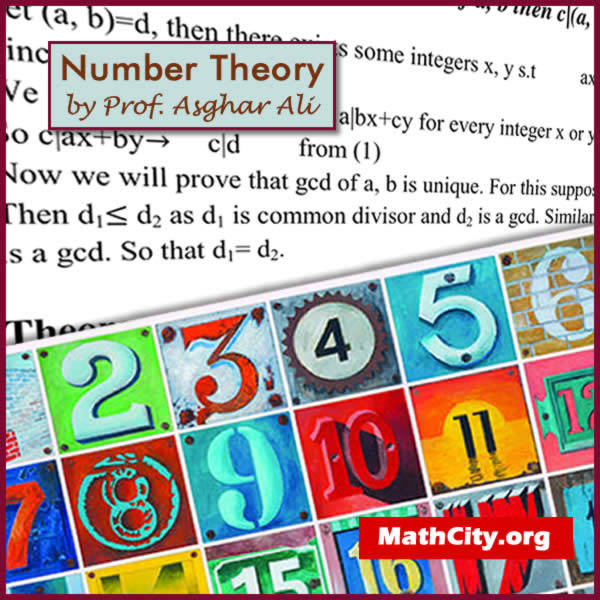# Number Theory by Prof. Asghar AliWe are very thankful to Prof. Asghar Ali for send these notes. These notes are very helpful to prepare BSc or ADS mathematics portion of Number Theory. Number theory is a subject in which students learn different concepts created on the set of integers. For example, the concept of divisibilty exists in the set of integer. Let a and b be any two integers suhc that $a\neq 0$. We say that a divides b denoted by a|b. when there exist integer c such that b = ca.

 Name Number Thoery Prof. Asghar Ali 34 pages PDF (see Software section for PDF Reader) 439 KB
• Divisibility
• Perfect number
• Division algorithm
• Mathematical induction
• G.C.D ( Greatest Common Divisor )or (H.C.F)
• Least Common Multiples(L.C.M)
• Prime numbers
• Fundamental theorem of arithematic
• Diophantine equation
• Polynomial theorem
• Congruences
• Properties of congruences
• Finding multiplicative inverses modulo n
• Chinese remainder theorem

• bsc/number-theory-by-prof-asghar-ali